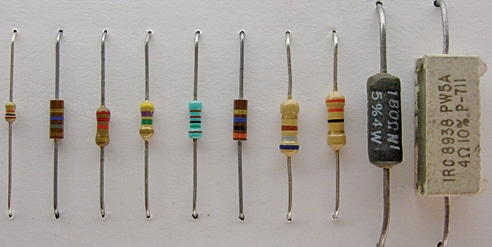# How Do You Determine The Resistor Values In A Circuit?

270Resistor values are selected after analysis for a given application. For most of the regular circuits, 1/4W resistors with ±5% tolerance carbon composition or carbon film resistors are used. However, for power applications, wire-wound, ceramic, and metal alloy resistors are commonly used.

A resistor of at least twice the calculated power dissipation is selected. If resistor is for power application, a resistor of at least three or four times higher than the calculated power dissipation value should be selected.

For commercial circuits or professionally designed circuits, the designer has to consider many other parameters as well while selecting a resistor for the circuit. It may involve scrutinising and analysing the datasheets provided by the manufacturer of the resistors.

There are several ranges of resistors available for electronics industry. The International Electrotechnical Commission is an international standards organisation that prepares and publishes international standards for all electrical, electronic, and related products including resistors. The standard range of resistors are grouped under E series : E3, E6, E12, E24, E48, E96, and E192.

For example, the standard resistances under E12 series are 10Ω, 12Ω, 15Ω, 18Ω, 22Ω, 27Ω, 33Ω, 39Ω, ….till 10MΩ. The values in the range are chosen such that they follow an exponential curve, and when you replace your calculated value with one from the preferred range, the error will be within a fixed percentage. Some resistors are colour coded to indicate the values or ratings while some resistors have the values printed with numbers or letters on the bodies.Different types of fixed resistors commonly used in the circuits are shown in Fig. 3. The most common process of selecting a resistor for a given application involves the following steps:

1. Selection of a resistor through a quick circuit analysis.
2. Checking the parameters like nominal value, tolerance, power rating, voltage drop, current through it, power dissipation by it and other properties, if required.
3. Calculating the power requirement of the desired resistor. A resistor of at least twice the calculated power dissipation should be selected. If it is a power application, the resistor of at least three or even four times the calculated power dissipation would be better.
4. Checking if the exact value of that resistor is available. If not, use parallel or series combination of resistors to get the required resistance value.
5. Deciding the type of resistor suitable for the given application or circuit. For example, through-hole or surface mount device (SMD), trimmable, variable, fixed type, etc.